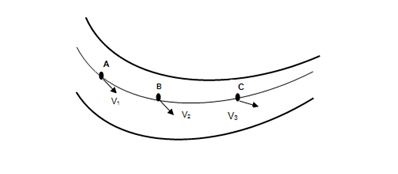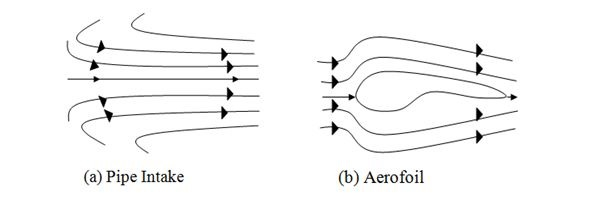# Streamline Flow

## Introduction

Each particle of fluid follows a single path while flowing under a particular and steady condition of the flow. Fluid velocity, low in value has no such turbulent velocity. No lateral mixing happens during that fluid motion. The particle’s motion is followed by an order in which the moving particles flow in a parallel and straight line.

## What is Streamline Flow?

An important laminar flow that is uninterrupted during flow and follows a straight and parallel line is streamline flow. This type of flow is mainly seen in part of a solid body which base is the main base to lead the flow in a parallel line. In a streamline flow, the direction of the particles remains the same within the near passage of time (Abbey et al. 2020). A smooth movement of layers is found in this flowing process. The speed of fluid depends on the velocity of the fluid, during flow. The characteristics of a streamline flow are dependent on the impacts of shear stress on the flow. In parallel to the liquid’s surface, the shear force works. During a streamline flow, gravitational force plays an important role.An atom’s path is regarded as the streamlined flow. A turbulent flow is different from a streamline flow.

## Types of Streamline Flow

There are mainly two types of streamline flow, which are laminar flow and turbulent flow. A type of flow in which the particles flow in a straight line and the nature is steady and smooth, the flow is regarded as laminar of streamline flow (Gajbhiye et al. 2020). In this type of fluid flow, no changes are found. Each and every layer of the flow is passed smoothly and with proper adjacent layers. In terms of low velocity, the fluid tends to mix laterally and mix with the layers (Warey, Han & Kaushik, 2021). At a higher velocity, the streamline flow turns into a turbulent flow. The range of viscosity is low in this type of flow.

Images Coming soon

An irregular motion is found in turbulent motion in which high velocities are associated. Unpredictable and random fluid particles go against the motion and create a chance of lateral mixing. There are three different and most important characteristics of turbulent flow which are apparent randomness, eddies and recirculation (Ströfer et al. 2018). Continuous changes in magnetic flow and direction are important characteristics of a turbulent flow which helps in understanding the nature of streamline flow. Laminar flow is disrupted and then turns into a turbulent flow. The changes in a streamline flow result in affecting the overall components and characteristic attributes of a streamline flow.

## Principles of Streamline Flow

Fluctuations in velocity are not seen in a streamline flow. It is a path of some imaginary particles that flow in a fluid. The streamline is always fixed in a straight line and maintains a regular path. Some of the most important properties such as pressure, and velocity are important as they affect the consistency of the flow (Saha, Biswas & Nath, 2020). There are some thin layers in a parallel flow of fluid that maintain consistency. Differences in speed and motion direction also are important components that impact on streamline.## Difference between Streamline Flow and Turbulent Flow

Turbulent Flow Streamline flow
In this flow type, the motion is chaotic in nature. A high lateral mixing is found in this type of liquid flow. In this type of flow, the motion is steady in nature.
The range of velocity in this type of flow is greater than the range of critical velocity. The range of velocity is less than the range of this flow’s critical velocity.
Each of the particles, including in a turbulent flow possesses a different speed. The directions of the particles are also different from each other. All particles, engaged in a streamline flow possess the same velocity. The direction and magnetic value are also the same for the particles.

## Application of Streamline Flow

• In understanding the length of a streamline, the concept of streamline flow is important.

• The direction of a particular fluid’s velocity is also determined by streamline flow.

• The fluid velocity range is tangential in nature that is related to the changes in time.

• Bernoulli's equation can be applied to an appropriate situation, where all the required conditions are met (Wang et al. 2020).

• The nature of a fluid flow can also be determined by streamline flow. Steady range, negligible viscosity and incompressible are important features.

## Summary

The dynamics of fluid are integrally connected to the range of classical mechanics. The behavior of a fluid’s flow is also integrally connected to this. The density of fluid remains the same and constant in a steady flow and the exact opposite nature is found in an unsteady flow. The paths, occupied by several fluid particles are regarded as the streamlines. As a parallel flow line is seen in a streamline flow, the velocity of the passing fluid particles remains the same with changes in time.

## FAQs

Q.1. What are the three different properties of a streamline flow?

Ans. Physical, kinematic, and thermodynamic properties are the three important properties of a streamline flow. The difference in composition creates changes in these properties.

Q.2. What are the significant characteristics of an ideal fluid?

Ans. An ideal fluid possesses no range of viscosity. This type of fluid is also suitable for compressing. The density of an ideal fluid is constant in nature.

Q.3. What happens to the viscosity of a liquid if the temperature increases?

Ans. In case of an increasing temperature, the range of a liquid’s viscosity reduces. As an example, a glass increases its viscosity when its surrounding temperature increases.

Q.4. Which formula is relevant to extract the value of Reynold’s number?

Ans. $\mathrm{Re=(\rho *v*L)/\mu }$ where p denotes the value of density. V represents the range of velocity and L signifies the value of a tube’s diameter. The value of viscosity is expressed by $\mathrm{\mu}$.

Updated on: 21-Dec-2022

237 Views